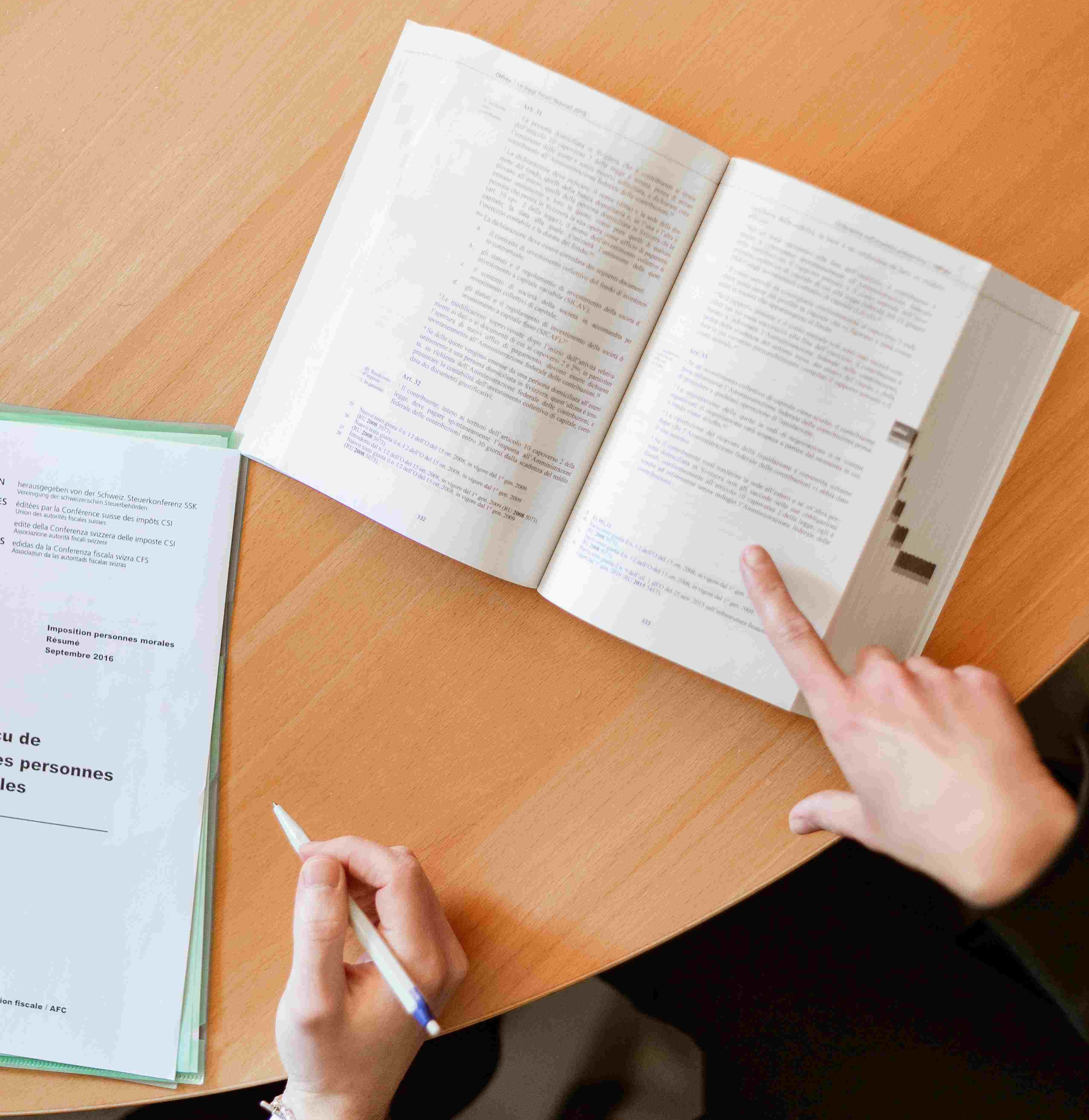# One‑Way ANOVA in SPSSOne Way ANOVA in SPSS One Way Analysis of Variance (ANOVA) is used to determine whether there are any statistically significant differences among the means of three or more independent groups.  One Way ANOVA has the following hypothesis. Null hypothesis: µ1 = µ2 = µ3  …µk (k is the number […]

# Logistic Regression in SPSSLogistic Regression in SPSS Logistic Regression is a supervised learning technique, which is used to understand the relationship between a dependent variable and one or more independent variables. Logistic regression is conducted by estimating the probabilities and by using the logistic regression equation. Assumptions 1. Logistic regression requires the dependent […]

# Linear Regression Analysis in SPSSLinear Regression Analysis in SPSS Linear Regression attempts to model the relationship between two variables by fitting a linear equation to the observed data. Linear Regression is a supervised learning algorithm. Here, we are predicting the dependent variable by using the independent variable. Assumptions 1. Dependent variable should be continuous. […]

# Independent Samples T Test using SPSSChi-Square test in SPSS Independent-Samples T Test is used to compare the means of two groups. The Independent Samples T Test is also known as Two Samples T Test. Independent Samples T Tests have the following hypotheses. Null hypothesis: The means of two populations are equal. Alternative hypothesis: The means […]

# Chi-Square test in SPSS